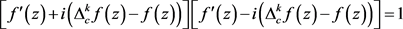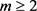# 一类费马型微分–差分方程解的问题Solution on Differential-Difference Equation of Fermat-Type

DOI: 10.12677/PM.2020.103023, PDF, HTML, XML, 下载: 177  浏览: 271  国家自然科学基金支持

Abstract: In this paper, we mainly discussed entire solutions with finite order of the following Fermat type differential-difference equation [f′(z)]2+[ Δckf(z)-f(z)]2=1 and obtained some interesting results.

1. 引言及主要结果,

$\underset{j=0}{\overset{k}{\sum }}{b}_{j}{a}^{j}=\frac{1}{i}$, $\underset{j=0}{\overset{k}{\sum }}{b}_{j}{\left(-a\right)}^{j}=-\frac{1}{i}$.

2012年，Liu等人  研究了以下费马型差分–微分方程，并获得了以下结论。

${\left[{f}^{\prime }\left(z\right)\right]}^{2}+{\left[f\left(z+c\right)-f\left(z\right)\right]}^{2}=1$. (1.1)

$f\left(z\right)=\mathrm{sin}\left(2z+Bi\right)/2$,

${\left[{f}^{\prime }\left(z\right)\right]}^{2}+{\left[{\Delta }_{c}^{k}f\left(z\right)-f\left(z\right)\right]}^{2}=1$. (1.2)

$f\left(z\right)\equiv ±1$，或者，其中 $a,b,d$ 是常数，并且满足 ${\text{e}}^{kac}={\left(-1\right)}^{k}$

${\left({\text{e}}^{ac}-1\right)}^{k}\ne 1$, $a=i{\left({\text{e}}^{ac}-1\right)}^{k}-i$.

$f\left(z\right)\equiv ±1$，或者

$f\left(z\right)=\frac{1}{3}\mathrm{sin}\left(3z+bi\right)+d$，其中 $b,d$ 是常数， $c=\left(\pi +n\pi \right)/3$

2. 一些引理

$\underset{i=1}{\overset{n}{\sum }}{f}_{i}\left(z\right){\text{e}}^{{g}_{i}\left(z\right)}\equiv 0$ ;

$T\left(r,{f}_{j}\right)=S\left(r,{\text{e}}^{{g}_{h}-{g}_{l}}\right)$, $r\to \infty$, $r\notin E$,

${f}_{i}\left(z\right)\equiv 0\left(1\le i\le n\right)$

$f\left(z\right)={z}^{k}P\left(z\right){\text{e}}^{Q\left(z\right)}$,

3. 定理3的证明

$f\left(z\right)$ 是方程(1.2)的有穷级整函数解，将(1.3)改写成下式。 (3.1)(3.2)

${f}^{\prime }\left(z\right)=\frac{{\text{e}}^{p\left(z\right)}+{\text{e}}^{-p\left(z\right)}}{2}$, (3.3)

${\Delta }_{c}^{k}f\left(z\right)-f\left(z\right)=\frac{{\text{e}}^{p\left(z\right)}-{\text{e}}^{-p\left(z\right)}}{2i}$. (3.4)

${\left[{\Delta }_{c}^{k}f\left(z\right)-f\left(z\right)\right]}^{\prime }=\underset{j=0}{\overset{k}{\sum }}{\left(-1\right)}^{k-j}{C}_{k}^{j}{f}^{\prime }\left(z+jc\right)-{f}^{\prime }\left(z\right)=\frac{{p}^{\prime }\left(z\right)}{2i}\left[{\text{e}}^{p\left(z\right)}+{\text{e}}^{-p\left(z\right)}\right]$. (3.5)

${f}^{\prime }\left(z+jc\right)=\frac{1}{2}\left[{\text{e}}^{p\left(z+jc\right)}+{\text{e}}^{-p\left(z+jc\right)}\right]$. (3.6)

$\underset{j=0}{\overset{k}{\sum }}{\left(-1\right)}^{k-j}{C}_{k}^{j}\left[{\text{e}}^{p\left(z+jc\right)}+{\text{e}}^{-p\left(z+jc\right)}\right]-\left[{\text{e}}^{p\left(z\right)}+{\text{e}}^{-p\left(z\right)}\right]=-i{p}^{\prime }\left(z\right)\left[{\text{e}}^{p\left(z\right)}+{\text{e}}^{-p\left(z\right)}\right]$. (3.7)

$\left[i{p}^{\prime }\left(z\right)-1+{\left(-1\right)}^{k}\right]\left[{\text{e}}^{p\left(z\right)}+{\text{e}}^{-p\left(z\right)}\right]+\underset{j=1}{\overset{k}{\sum }}{\left(-1\right)}^{k-j}{C}_{k}^{j}\left[{\text{e}}^{p\left(z+jc\right)}+{\text{e}}^{-p\left(z+jc\right)}\right]=0$. (3.8)，则对任意的 $0\le j，均有

$\rho \left({\text{e}}^{p\left(z+jc\right)-p\left(z+lc\right)}\right)=m-1\ge 1$, 且 $\rho \left({\text{e}}^{p\left(z+jc\right)+p\left(z+lc\right)}\right)=m\ge 2$.

(3.8)式两边同时乘以 ${\text{e}}^{p\left(z\right)}$，并化简，可得

$\left[ia-1+{\left(-1\right)}^{k}\right]\left[{\text{e}}^{2p\left(z\right)}+1\right]+\underset{j=1}{\overset{k}{\sum }}{\left(-1\right)}^{k-j}{C}_{k}^{j}\left[{\text{e}}^{p\left(z\right)+p\left(z+jc\right)}+{\text{e}}^{p\left(z\right)-p\left(z+jc\right)}\right]=0$.

$\left[ia-1+{\left(-1\right)}^{k}\right]\left[{\text{e}}^{2p\left(z\right)}+1\right]+\underset{j=1}{\overset{k}{\sum }}{\left(-1\right)}^{k-j}{C}_{k}^{j}\left[{\text{e}}^{2p\left(z\right)+ajc}+{\text{e}}^{-ajc}\right]=0$. (3.9)

${\text{e}}^{2p\left(z\right)}\left[\underset{j=0}{\overset{k}{\sum }}{\left(-1\right)}^{k-j}{C}_{k}^{j}{\text{e}}^{ajc}+ia-1\right]+\left[\underset{j=0}{\overset{k}{\sum }}{\left(-1\right)}^{k-j}{C}_{k}^{j}{\text{e}}^{-ajc}+ia-1\right]=0$. (3.10)

$\left\{\begin{array}{l}\underset{j=0}{\overset{k}{\sum }}{\left(-1\right)}^{k-j}{C}_{k}^{j}{\text{e}}^{ajc}+ia-1=0;\\ \underset{j=0}{\overset{k}{\sum }}{\left(-1\right)}^{k-j}{C}_{k}^{j}{\text{e}}^{-ajc}+ia-1=0.\end{array}$

${\text{e}}^{2p\left(z\right)}=-\frac{\underset{j=0}{\overset{k}{\sum }}{\left(-1\right)}^{k-j}{C}_{k}^{j}{\text{e}}^{-ajc}+ia-1}{\underset{j=0}{\overset{k}{\sum }}{\left(-1\right)}^{k-j}{C}_{k}^{j}{\text{e}}^{ajc}+ia-1}$,

$\left\{\begin{array}{l}{\left({\text{e}}^{ac}-1\right)}^{k}+ia-1=0;\\ {\left({\text{e}}^{-ac}-1\right)}^{k}+ia-1=0.\end{array}$ (3.11)

$f\left(z\right)=\frac{{\text{e}}^{az+b}-{\text{e}}^{-az-b}}{2a}+d=\frac{1}{ai}\mathrm{sin}\left[aiz+bi\right]+d$.

  Hayman, W.K. (1964) Meromorphic Functions. Clarendon Press, Oxford.  Yang, L. (1993) Value Distribution Theory. Springer-Verlag, Berlin.  Yi, H.X. and Yang, C.C. (2003) Uniqueness Theory of Meromorphic Functions. Science Press, Beijing.  Gross, F. (1966) On the Equation fn + gn = 1. Bulletin of the American Mathematical Society, 72, 86-88. https://doi.org/10.1090/S0002-9904-1966-11429-5  Gross, F. (1966) On the Equation fn + gn = hn. The American Mathematical Monthly, 73, 1093-1096. https://doi.org/10.2307/2314644  Yang, C.C. and Li, P. (2004) On the Transcendental Solutions of a Certain Type of Nonlinear Differential Equations. Archiv der Mathematik, 82, 442-448. https://doi.org/10.1007/s00013-003-4796-8  Chen, M.F., Gao, Z.S. and Du, Y.F. (2017) Existence of Entire Solutions of Some Non-Linear Differential-Difference Equations. Journal of Inequalities and Applications, 90, 17 p. https://doi.org/10.1186/s13660-017-1368-1  Chiang, Y.M. and Feng, S.J. (2008) On the Nevanlinna Characteristic of f(z+η) and Difference Equations in the Complex Plane. The Ramanujan Journal, 16, 105-129. https://doi.org/10.1007/s11139-007-9101-1  Laine, I., Rieppo, J. and Silvennoinen, H. (2005) Remarks on Complex Difference Equations. Computational Methods and Function Theory, 5, 77-88. https://doi.org/10.1007/BF03321087  Halburd, R.G. and Korhonen, R.J. (2006) Difference Analogue of the Lemma on the Logarithmic Derivative with Applications to Difference Equations. Journal of Mathematical Analysis and Applications, 314, 477-487. https://doi.org/10.1016/j.jmaa.2005.04.010  Liu, K., Cao, T.B. and Cao, H.Z. (2012) Entire Solutions of Fermat Type Differential-Difference Equations. Archiv der Mathematik, 99, 147-155. https://doi.org/10.1007/s00013-012-0408-9  Liu, K. (2015) Fermat Type Differential and Difference Equations. Electronic Journal of Differential Equations, 159, 1-10. https://doi.org/10.1186/s13662-015-0534-3  Gao, L.Y. (2017) On Entire Solutions of Two Types of Systems of Complex Differential-Difference Equation. Acta Mathematica Scientia, 37B, 187-194. https://doi.org/10.1016/S0252-9602(16)30124-2  高凌云. 关于一类复微分-差分方程组的解[J]. 数学年刊, 2017, 38A(1): 23-30.  Gao, L.Y. (2016) Entire Solutions of Two Types of Systems of Complex Differential-Difference Equations. Acta Mathematica Scientia, 59, 677-684.